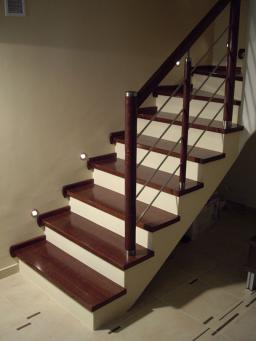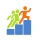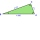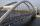# Stairway

Stairway has 20 steps. Each step has a length of 22 cm and a height of 15 cm. Calculate the length of the handrail of staircases if on the top and bottom exceeds 10 cm.

x =  552.5411 cm

### Step-by-step explanation:Did you find an error or inaccuracy? Feel free to write us. Thank you!Tips to related online calculators
Pythagorean theorem is the base for the right triangle calculator.

#### You need to know the following knowledge to solve this word math problem:

We encourage you to watch this tutorial video on this math problem:

## Related math problems and questions:

• StairwayWhat angle rising stairway if step height in 20 cm and width 26 cm?
• Speed of Slovakian trainsRudolf decided to take the train from the station 'Ostratice' to 'Horné Ozorovce'. In the train timetables found train Os 5409 : km 0 Chynorany 15:17 5 Ostratice 15:23 15:23 8 Rybany 15:27 15:27 10 Dolné Naštice 15:31 15:31 14 Bánovce nad Bebravou 15:35 1
• IS trapezoidCalculate the length of diagonal u and height v of isosceles trapezoid ABCD, whose bases have lengths a = |AB| = 37 cm, c = |CD| = 29 cm and legs b = d = |BC| = |AD| = 28 cm.
• Body diagonalCalculate the length of the body diagonal of a block with dimensions: a = 20 cm, b = 30 cm, c = 15 cm.
• Rectangular triangle PQRIn the rectangular triangle PQR, the PQ leg is divided by the X point into two segments of which longer is 25cm long. The second leg PR has a length 16 cm. The length of the RX is 20 cm. Calculate the length p of side RQ. The result is round to 2 decimal
• CalculateCalculate the height to the base of the isosceles triangle ABC if the length of the base is c = 24cm and the arms have a length b = 13cm.
• StepsFind the height between the two floors if you know that the number of steps between the two floors is 18, the gradient is 30º, and the length of the step is 28.6 cm. Report the result in centimeters to the nearest centimeter.
• Medians in RTThe rectangular triangle ABC has a length of 10 cm and 24 cm. Points P, Q, R are the centers of the sides of this triangle. The perimeter of the PQR triangle is:
• Center of gravityIn the isosceles triangle ABC is the ratio of the lengths of AB and the height to AB 10:12. The arm has a length of 26 cm. If the center of gravity T of triangle ABC find area of triangle ABT.
• Two chordsIn a circle with radius r = 26 cm two parallel chords are drawn. One chord has a length t1 = 48 cm and the second has a length t2 = 20 cm, with the center lying between them. Calculate the distance of two chords.
• ArmCalculate the length of the arm r of isosceles triangle ABC, with base |AB| = 14 cm and a height v=18 cm.
• Median in right triangleIn the rectangular triangle ABC has known the length of the legs a = 15cm and b = 36cm. Calculate the length of the median to side c (to hypotenuse).
• StepsPatrick step is 65 cm long, and his dad step is 10 cm longer. Dad goes bridge in 52 steps. How many steps will go the same bridge by Patrick?
• WalkersWalker, which makes 120 steps per minute, make distance from point A to point B for 55 minutes. The length of his step is 75cm. For how long does this distance go boy who will do 110 steps 60 cm long in a minute?
• Right isoscelesCalculate area of the isosceles right triangle which perimeter is 26 cm.
• Perimeter of circleCalculate the circumference of described circle to the triangle with sides 9,12,15 cm.
• Pyramid heightFind the volume of a regular triangular pyramid with edge length a = 12cm and pyramid height h = 20cm.